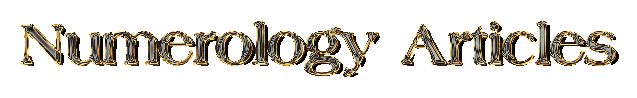www.richardking.netThe Nature (also referred to as Natural Nature) is the personality and manner in which an individual goes about doing things. It is derived from the consonants in the full natal name. To determine the Nature,  simply place the numerical value of each consonant below it, add left to right and reduce to a single digit. Then refer to the Keywords to associate the number with its characteristics.

 1 2 3 4 5 6 7 8 9 A B C D E F G H I J K L M N O P Q R S T U V W X Y Z

Example #1 - John James Doe: Born: 13 March 1990: Nature is a 6 (Six)

 J O H N J A M E S D O E 1 8 5 1 4 1 4 = 24 > 2 + 4 = 6 > Nature

Computing the Material Nature Number

The Material Nature can be considered as the personality manifesting in the material world. To determine the Material Nature, simply add the Simple Nature to the Simple Lifepath.

JJD Lifepath of 8 plus Nature of 6 = Material Nature of 5 (14 > 1 + 4 = 5)

Example #2: Linda Leigh Smith: Born: 26 September 1998 : Natural Nature is a 9 (Nine)

 L I N D A L E I G H S M I T H 3 5 4 3 7 8 1 4 2 8 = 45 > 4 + 5 = 9 > Nature

Material Nature

LLS Lifepath of 8 plus Nature of 9 = Material Nature of 8 (8 + 9 = 17 > 1 + 7 = 8)